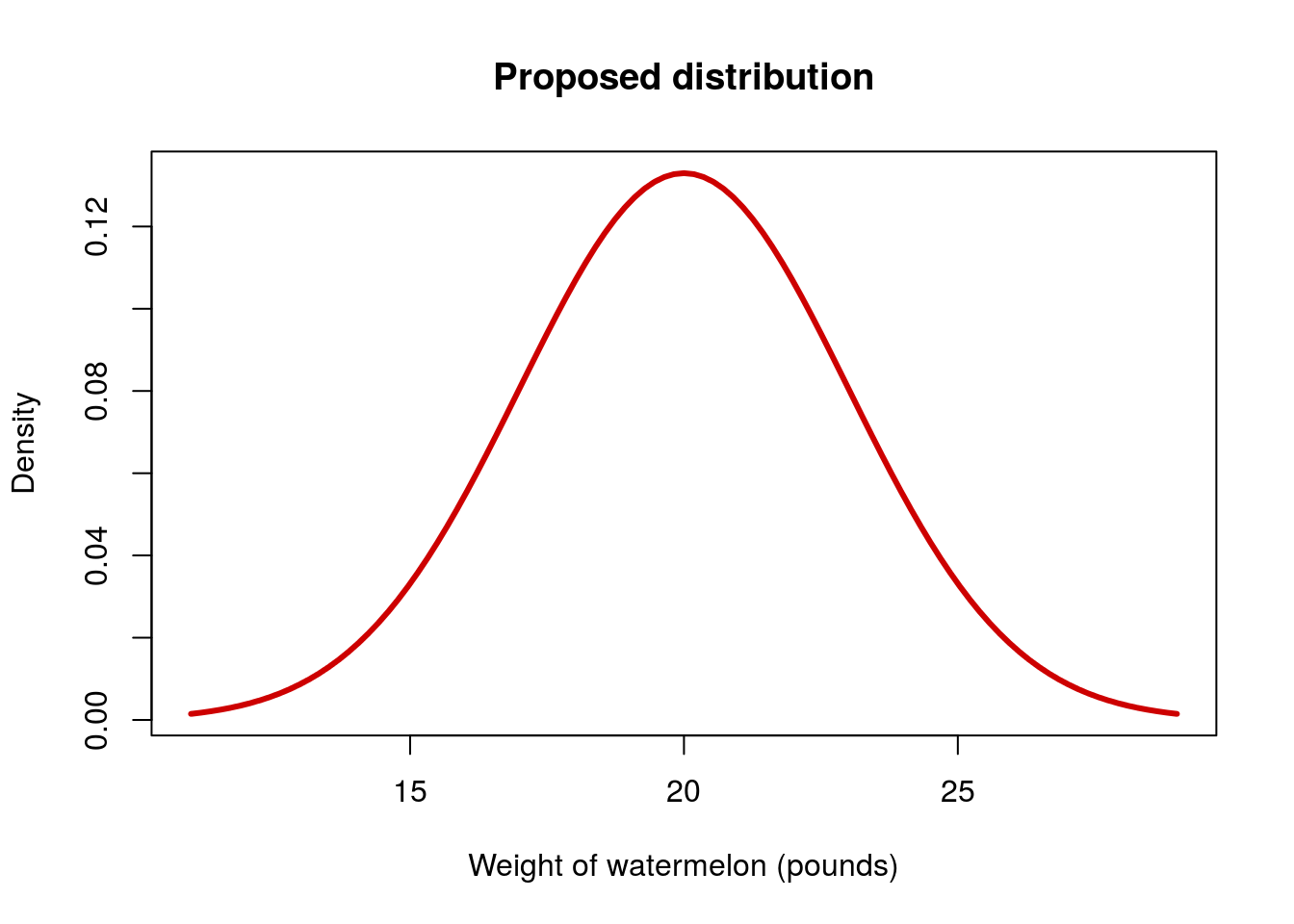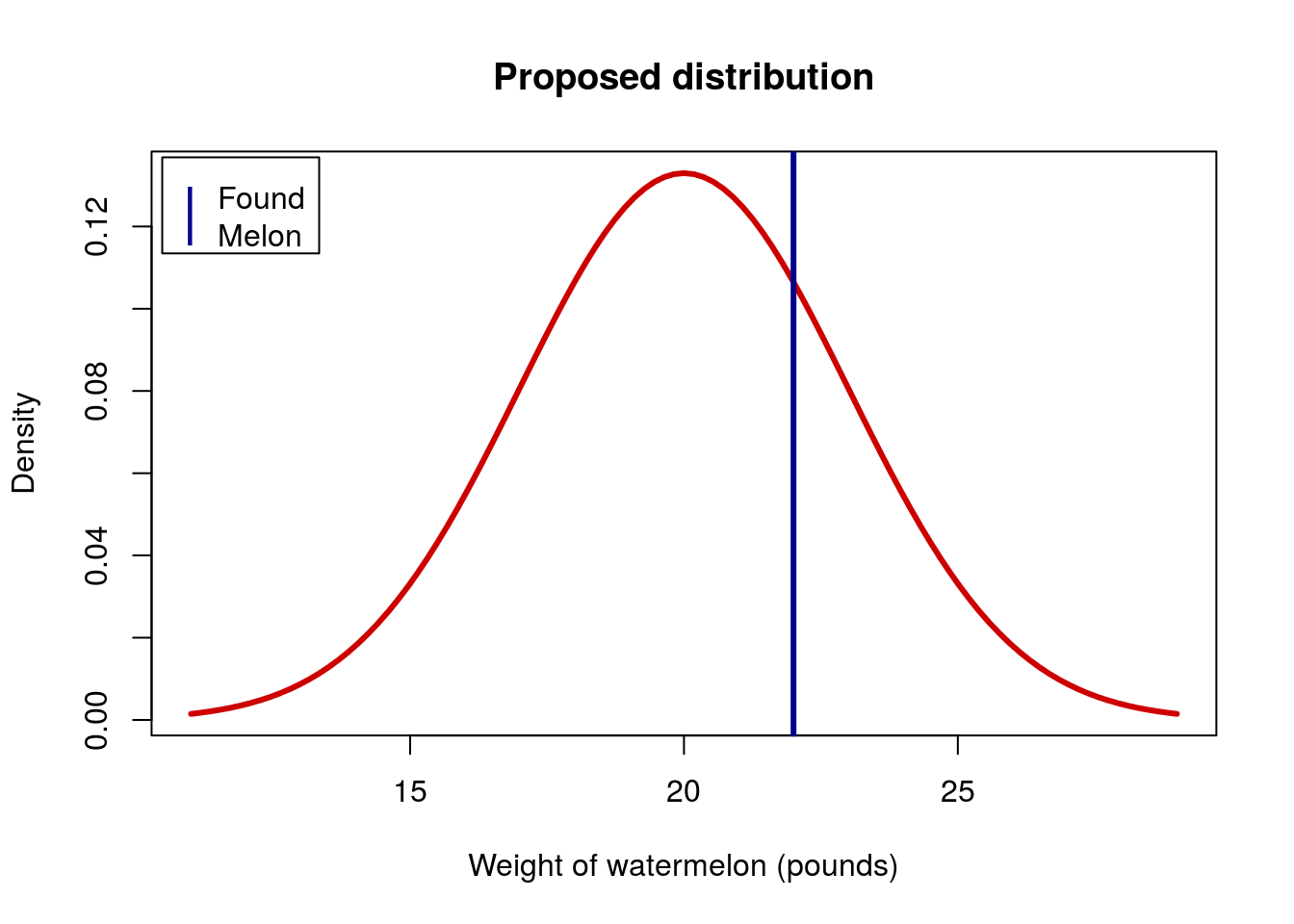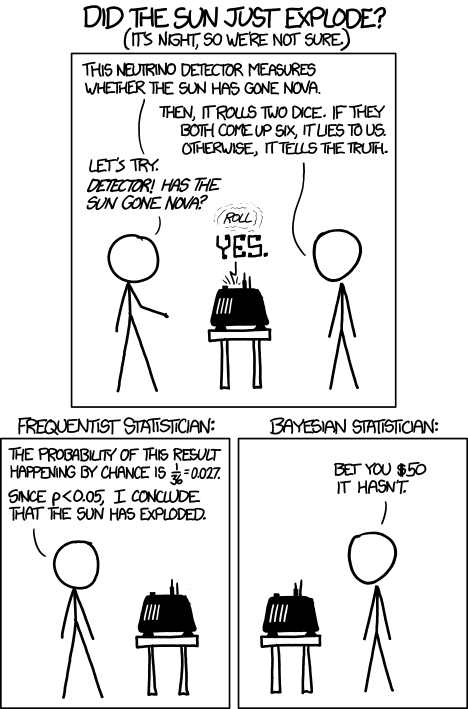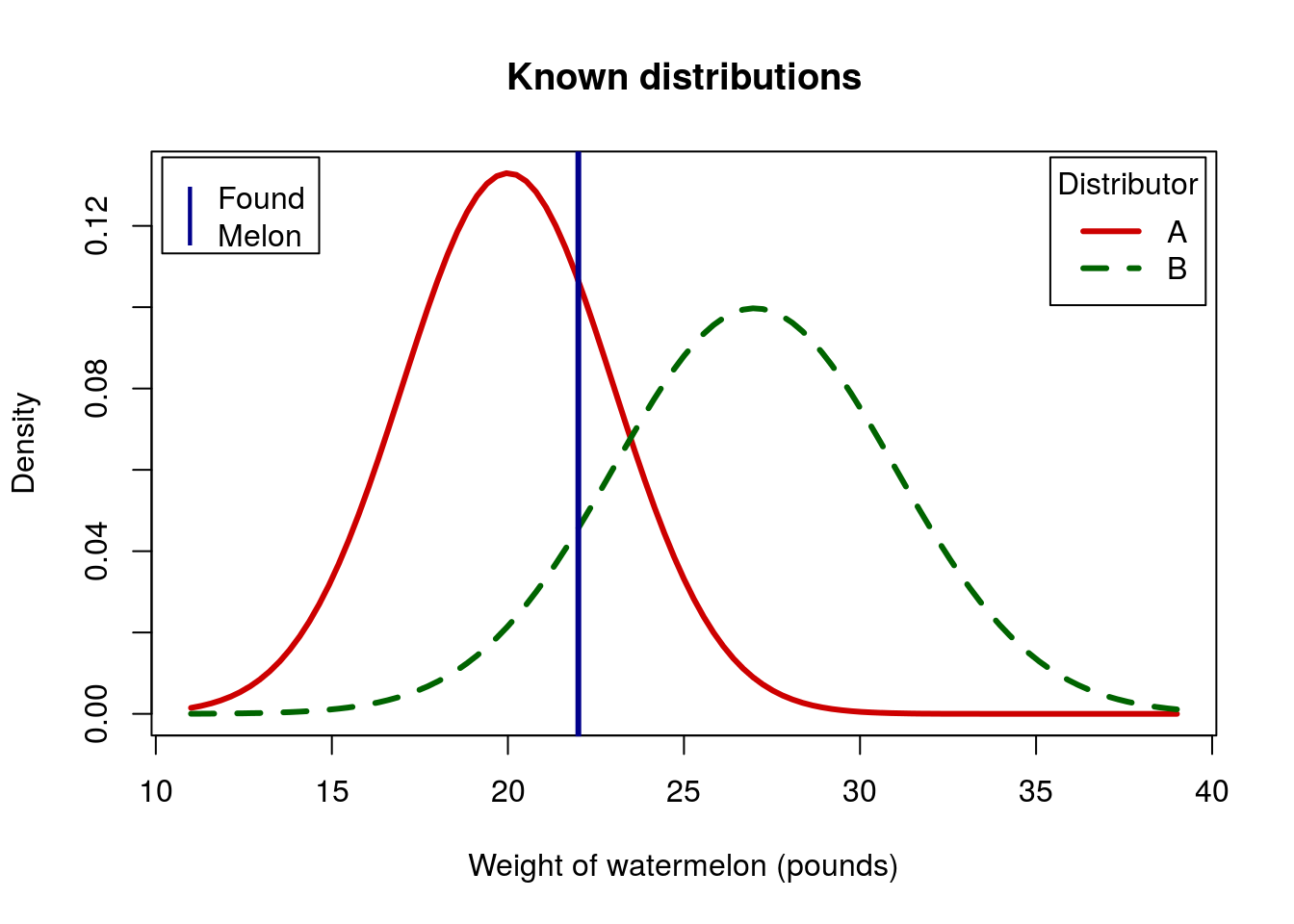A p-value is defined as:

A p-value is the probability of generating data that are as extreme as, or more extreme than, your collected data, given that the null hypothesis was true.

However, there are a number of common misconceptions about how a p-value can be interpretted. Even an Editor in Nature implictly got it wrong – these are incredibly common. However, it is important to get this right. The most common misconceptions seems to be that the p-value is the probability that the null is true. This is incorrect, though an understandable mistake. The correction (particularly to such a wordy definition like above) may seem pedantic. However, this misconception is not just an over simplification. It is just incorrect.

What follows is a simplified example that hopefully shows the distinction between these two things. At the end, I have included a simplified example of the process that would be necessary to make a statement similar to that one.

# An example

Imagine a supermarket that carries watermelons. I tell you that the average weight of the watermelons is 20 pounds and that they have a standard deviation of 3 pounds. We can see this distribution here:You aren’t sure whether or not I am telling the truth. So, you pick up a single watermelon and find that it weighs 22 pounds. What can you conclude about the proposed distribution? Well, we can start by adding a line to the plot showing it:That doesn’t seem like a particularly odd melon to have found if I am telling the truth. We can see exactly how odd by finding the probability of finding a watermelon that large or larger if my proposed distribution is true (I used R, but you could use a table). This shows that 25.2% of watermelons should be 22 pounds or heavier. Because we did not have an a priori directional test, we double this to get a two-tailed p-value of 0.504.

Does this mean that there is a 50.4% chance that the null is true?

No, all it means is that there was a 50.4% chance of getting a melon this extreme or more extreme. Given that we are not surprised by the size of this melon, we would have no reason to doubt my proposed distribution. This means that it would remain plausible, but that it is also possible that the true average is something different.

If, on the other hand, we had picked up a watermelon weighing 35 pounds, we would be very surprised. We would likely conclude that such a melon is unlikely to be found by chance if the proposed distribution is correct, so we would say that we no longer believed it to be true.

# Connection to hypothesis testing

This is exactly the same process as statistical test. The only difference here is that I am specifying the standard deviation in addition to the mean. This allows a clarifying example with a single watermelon, which is generally easier to understand than complicated formulas for standard errors and sampling distributions.

In the case of the 22 pound watermelon, we would say we fail to reject the null, though that does not necessarily mean the null is true.

In the case of the 35 pound watermelon, we would reject the null, though it does not tell us what the true mean should be.

Neither of these give us a probability that the null is true. This may seem like a nuance, but it is in reality an important distinction. Here is a good comic illustrating the problems with this type of conclusion:xkcd Frequentists vs. Bayesians

In this comic, the equivalent statement would be that there is a 97.2% chance that the sun went nova (only a 2.8% chance that the null of no nova is true).

Below is an example of the types of additional information that are needed in order to draw conclusions about the probability that a particular hypothesis is true.

# Giving a probability that the null is true

Often we want to be able to say something along the lines of “The probability that the null is true.” A p-value does not allow us to say that, though Bayesian analysis can under certain circumstances. For more on Bayes (including the math), see the full lecture notes. Here, all that is important to know is that it compares various proposed distributions. The case below is simplified, but should illustrate the point.

Now, instead of a proposed distribution, imagine that we have perfect information. The manager tells us that the store uses exactly two suppliers of watermelons. They get an equal number of watermelons from each, and then the watermelons are mixed together.

• The melons from Distributor A have an average of 20 pounds and a standard deviation of 3 pounds (the null from above).
• The melons from Distributor B have an average of 27 pounds and a standard deviation of 4 pounds (we can think of this like a specific alternative hypothesis, like for power analysis).

Now, when you find your 22 pound watermelon, your question is not “Is the null likely?” but rather: “which distributor is more likely?”

We can visualize these together (note that the area under each curve is 1; the curve for Distributor B is just wider):As you can see, a 22 pound watermelon is more likely to be from Distributor A. We can use the density functions for each distributor to show that 70% of 22 pound watermelons should be from Distributor A.

This can be made more clear if we think a bit more concretely. Let’s imagine that 1,000 watermelons came in from each distributor. Further, let’s imagine that we are only weighing to the nearest pound. That is, anything between 21.5 and 22.5 pounds will count as a 22 pound watermelon.

Given the distributions, we would expect that 106 of the watermelons from Distributor A and 46 of the watermelons from Distributor B would be counted as 22 pound watermelons. So, if you pick up a 22 pound watermelon, we can determine the probability that it came from Distributor A as:

$\textrm{P(Dist. A)} = \frac{\textrm{number of 22 pound melons from A}}{\textrm{total number of 22 pound melons}} = \frac{106}{106 + 46} = \frac{106}{152} = 0.7$

Using either of these approaches, we can say that, given we picked up a 22 pound watermelon, there is a 70% chance that it came from Distributor A.

This is similar to the desire to give a probability that the null is true, but note that it required a very specific alternative hypothesis to do so.

I should note here as well, that the total number of watermelons from each distributor matters greatly. I used the simple example of equal numbers above, but it is clear to see that if we change those numbers, the probabilities change. As an illustration, imagine that next week, while Distributor A delivers 1,000 watermelons, Distributor B delivers 4,000. Now, we expect 4 times as many 22 pound watermelons from B as we got last time (183 this time). That means our equation for the probability that it came from Distributor A is now:

$\textrm{P(Dist. A)} = \frac{\textrm{number of 22 pound melons from A}}{\textrm{total number of 22 pound melons}} = \frac{106}{106 + 183} = \frac{106}{289} = 0.37$

This means that now, given that we picked up the same 22 pound watermelon, there is only a 37% chance that it came from Distributor A.

That initial information is called the “prior” in Bayesian analysis, and is incredibly important. Without it, you can not calculate the probability that either proposed distribution is true.

This is another reason why the statement that the “p-value is the probability that the null is true” is incorrect. Without specifying the probability that the null was true before running the test (and specifying a specific alternative or set of alternatives) you don’t have enough information to make that statement.

If we return to the nova example above, we might specify the prior probability that the sun has gone nova as 1 in a million (though even that is likely too high). Our equation for the probability that the sun as gone nova is then:

$\textrm{P(Nova)} = \frac{\textrm{probability of nova and not two sixes}}{\textrm{probability of nova or two sixes}} =$

$\frac{\frac{1}{1,000,000} \times \frac{35}{36}}{\frac{1}{1,000,000} \times \frac{35}{36} + \frac{999,999}{1,000,000} \times \frac{1}{36}} = 0.000035$

That is, the probability that the sun has gone nova, given those assumptions is about 1/28572 - substantially less than the 1/36 supposed if you merely use the p-value.